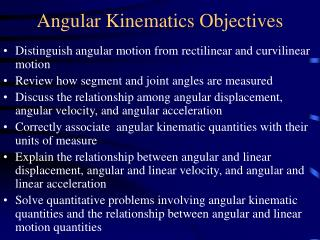Download PresentationAngular Kinematics Objectives

Angular Kinematics Objectives - PowerPoint PPT Presentation

Angular Kinematics Objectives. Distinguish angular motion from rectilinear and curvilinear motion Review how segment and joint angles are measured Discuss the relationship among angular displacement, angular velocity, and angular accelerationI am the owner, or an agent authorized to act on behalf of the owner, of the copyrighted work described.
Download PresentationAngular Kinematics Objectives

Download Policy: Content on the Website is provided to you AS IS for your information and personal use and may not be sold / licensed / shared on other websites without getting consent from its author.While downloading, if for some reason you are not able to download a presentation, the publisher may have deleted the file from their server.

- - - - - - - - - - - - - - - - - - - - - - - - - - E N D - - - - - - - - - - - - - - - - - - - - - - - - - -
Presentation Transcript
1. Angular Kinematics Objectives • Distinguish angular motion from rectilinear and curvilinear motion • Review how segment and joint angles are measured • Discuss the relationship among angular displacement, angular velocity, and angular acceleration • Correctly associate angular kinematic quantities with their units of measure • Explain the relationship between angular and linear displacement, angular and linear velocity, and angular and linear acceleration • Solve quantitative problems involving angular kinematic quantities and the relationship between angular and linear motion quantities

2. Angular Kinematics Outline • Reading assignment: Chapter 11 • Measuring angles • Relative (joint) vs absolute (segment) angles • Measuring angles • Goniometer (relative, or joint angles) • Inclinometer (absolute, or segment angles) • Electrogoniometer • Analytic calculation from 3 points in space • Angular displacement • Units of measurement (degrees, radians, revolutions) • Relationship between linear and angular motion • Linear and angular acceleration • Self-study problems: • Introductory problems, pp 376-377: 4,5,7,10 • Homework problems (due Tuesday, April 11): • Additional problems, p 377-378: 1,10

3. Measuring joint & segment angles Joint centers form Vertices for jt angles Segment angles Joint angles

4. Measuring angles of bodily segments Leighton flexometer (absolute, or stegment angles) Goniometer (relative, or joint angles)

5. Units of angular measurement Degrees Radians Revolutions

6. Relationship between linear and angular motion • Would you rather have a long or short baseball bat or tennis racquet? (sample problem 2, p 372)

7. Calculating linear Velocity when Angular velocity & rotation radius Are known

8. Tangential and radial acceleration Tangential acceleration represents change in magnitude of velocity Sample prob #3, p 375 Radial acceleration represents change in direction

9. Calculating tangential acceleration, Radial acceleration, and resultant acceleration